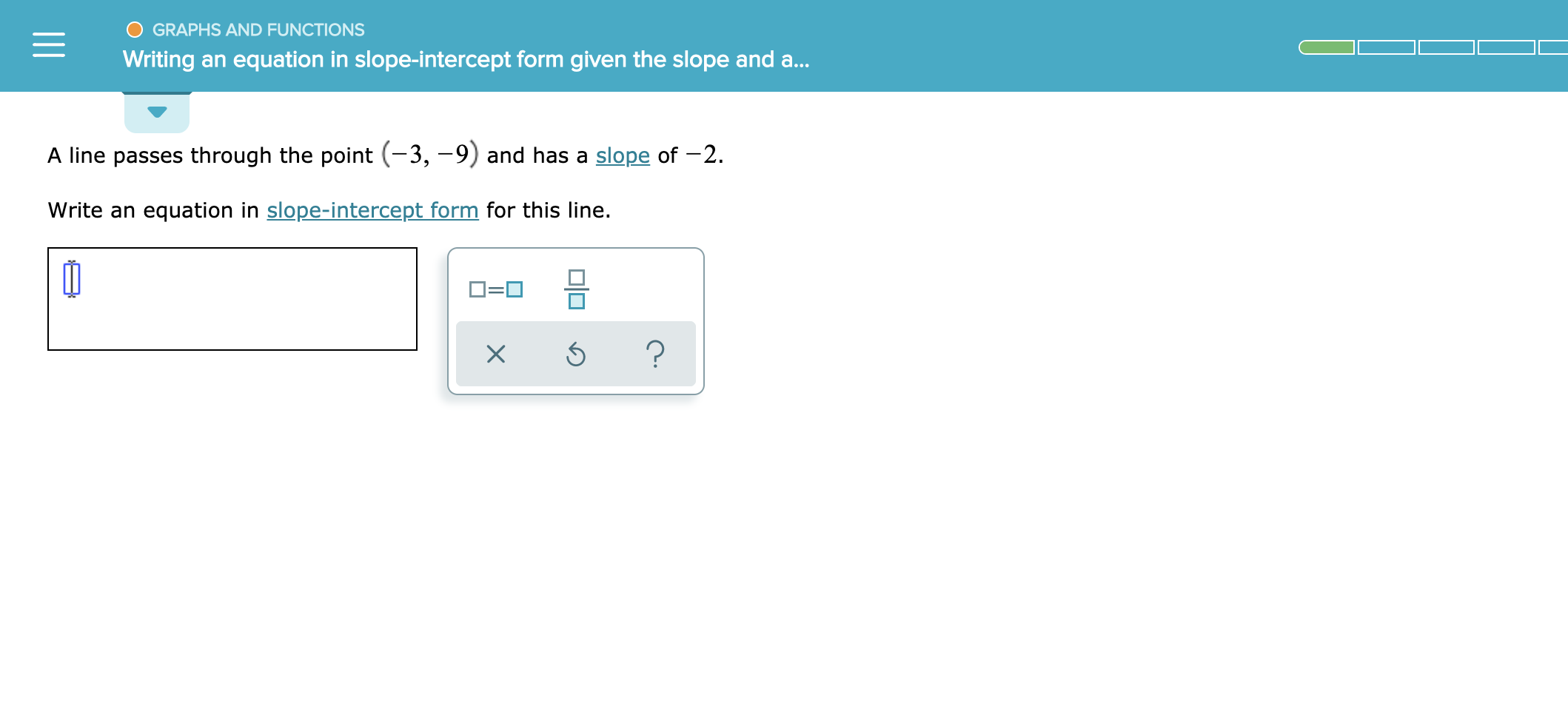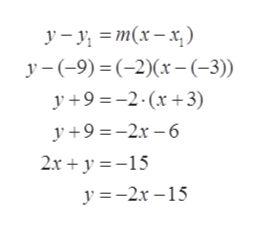# GRAPHS AND FUNCTIONSWriting an equation in slope-intercept form given the slope and a..A line passes through the point (-3, -9) and has a slope of -2.Write an equation in slope-intercept form for this line.?X

Question
3 views

See attachedhelp_outlineImage TranscriptioncloseGRAPHS AND FUNCTIONS Writing an equation in slope-intercept form given the slope and a.. A line passes through the point (-3, -9) and has a slope of -2. Write an equation in slope-intercept form for this line. ? X fullscreen
check_circle

Step 1

To determine the line passes through the point (-3, -9) and slope m is -2.

Step 2

Equation of line passes through the point (x1, y1) and slope m is given as

Step 3

Plug the value of x1, y1, m in the ...help_outlineImage Transcriptionclosey-y = m(x-x) y-9) (-2)(x- (-3)) y 9-2 x3) y 9-26 2x 15 y 2r-15 fullscreen

### Want to see the full answer?

See Solution

#### Want to see this answer and more?

Solutions are written by subject experts who are available 24/7. Questions are typically answered within 1 hour.*

See Solution
*Response times may vary by subject and question.
Tagged in

### Calculus